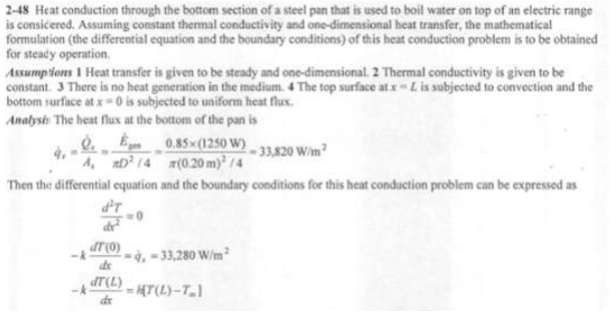heat and mass transfer problems engineering equations heat and mass transfer school homework engineering heat and mass transfer formulas heat and mass transfer solutions to heat and mass transfer problems full solution engineering problem solution heat and mass transfer math problems engineering equations heat and mass transfer school homework engineering solutions to heat and mass transfer formulas heat problems mass problem solutions to transfer problems full solution heat and mass transfer
heat and mass transfer problems engineering equations heat and mass transfer school homework engineering heat and mass transfer formulas heat and mass transfer solutions to heat and mass transfer problems full solution engineering problem solution heat and mass transfer math problems engineering equations heat and mass transfer school homework engineering solutions to heat and mass transfer formulas heat problems mass problem solutions to transfer problems full solution heat and mass transfer
Highalphabet Home Page heat and mass transfer problem solutions Heat and Mass Transfer Page
Consider a steel pan used to boil water on top of an electric range. The bottom section of the pan is L=0.3 cm thick and has a diameter of D=20cm. The electric heating unit on the range top consumes 1250 W of power during cooking, and 85 percent of the heat generated in the heating element is transferred uniformly to the pan. Heat transfer from the top surface of the bottom section to the water is by convection with a heat transfer coefficient of h. Assuming constant thermal conductivity and one-dimensional heat transfer, express the mathematical formulation (the differential equation and the boundary conditions) of this heat conduction problem during steady operation. Do not solve.Consider a steel pan used to boil water on top of an electric range. The bottom section of the pan is L=0.3 cm thick and has a diameter of D=20cm. The electric heating unit on the range top consumes 1250 W of power during cooking, and 85 percent of the heat generated in the heating element is transferred uniformly to the pan. Heat transfer from the top surface of the bottom section to the water is by convection with a heat transfer coefficient of h. Assuming constant thermal conductivity and one-dimensional heat transfer, express the mathematical formulation (the differential equation and the boundary conditions) of this heat conduction problem during steady operation. Do not solve.